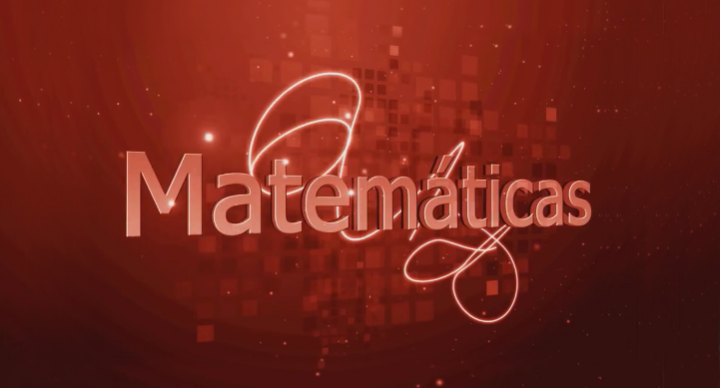# Mathematics Course 1 – Spanish### Course Overview

Mathematics Course 1 - Spanish, taught by Jaritza Sierra, is for Spanish-speaking students. This course introduces English concepts, preparing Spanish-speaking students to transition to the English-speaking classroom. During this course, students will study ratios and rates, practice solving problems with negative integers, use expressions and equations to solve problems, and develop an understanding of statistical thinking. Some schools utilize this curriculum at the 6th grade level.

### Sample Lesson - Evaluating Algebraic ExpressionsThis course was developed by the International Academy of Science. Learn More

### Scope and Sequence

Unit 1 – Whole Numbers, Decimals, Prime Factorization, and Exponents This unit discusses comparing and ordering whole numbers and decimals, followed by adding, subtracting, and multiplying decimals, as well as dividing whole numbers and decimals.  It goes on to cover prime factorization and exponents. Unit 2 – GCF, LCM, Fractions, and Mixed Numbers In this unit, students learn about greatest common factors and least common multiples.  They go on to study fractions and mixed numbers, including ordering proper fractions, mixed numbers, and multiplying and dividing fractions with mixed numbers. Unit 3 – Integers While studying this unit, students learn about integers, including the number line, adding, subtracting, multiplying and dividing integers, and integers in the coordinate plane.  They also study distance and reflections. Unit 4 – Equations In this unit students begin to understand concepts of algebra.  They begin with order of operations and continue with evaluating algebraic expressions, properties of operations, equations, and solutions of equations.  Going on, they study addition and subtraction equations, followed by multiplication and division equations. Unit 5 – Inequalities, Relationships, and Variables This unit presents students with concepts about inequalities, relationships, and variables.  Students begin with representing situations with inequalities and solving one-step inequalities.  Next, they learn to represent relationships, and finally they study dependent and independent variables. Students are presented with the Mid-Term Review and Exam. Unit 6 – Ratios and Percents This unit focuses on ratios and percents.  Students learn about ratios in proportional relationships, solving problems using ratios and rate, and using models to understand percents.  Next they study percents, fractions, and decimals, followed by applying percents. Unit 7 – Geometry In this unit, students study basic concepts of geometry.  They begin with properties of triangles, side lengths, and angle measures.  They continue with quadrilaterals and go on to perimeter and area of composite figures.  Next students study area of triangles, trapezoids, and polygons, followed by polygons on the coordinate plane, nets and surface area, and volume. Unit 8 – Statistics This unit discusses concepts of statistics.  Students learn about types of variables and graphs, measures of the center, median, and measures of variability.  Next they study interquartile range, and finish the unit with box plots and histograms. Students are presented with the Final Review and Exam.
This course does not have any sections.